DENSITY BASICS
To begin our discussion of density, we look first at density and specific gravity definitions for gases, liquids, and solids, and later at the density of mixtures. After that, we look at how a remote sensing tool, such as a borehole compensated density log measures density, without being able to weigh or measure the volume of a rock, followed by a review of how the photoelectric log is measured by the same logging tool.

The terms specific gravity and density are often used interchangeably and this is incorrect. Density is weight per unit volume and has units. These units are generally pounds per cubic foot, grams per cubic centimeter or Kilograms per cubic meter. Specific gravity is the ratio of the weight of a given volume of material to the weight of the same volume of water, and is unitless.

In reservoir engineering, density and specific gravity are expressed in several manners. The first is the common expression relating weight to volume:
1: DENSl = W / V

Where:
DENSl = density (lb/cuft or gm/cc)
V = volume (cu/ft or cc)
W = weight (pounds or grams)

A useful expression for conversion purposes is:
2. Density in lb/cu.ft. = 62.4 times density in gm/cc

The specific gravity of oil is often given on the API gravity scale and must be converted to density:
3: DENSl = 8829.6 / (131.5 + SGapi)
OR: 3A: DENSk = 141500 / (131.5 + SGapi)

Where:
DENSl = density (lb/cuft)
DENSk = density (kg/m3)
SGapi = specific gravity of oil (API degrees)

The specific gravity of gas is often related to the weight of air, which is defined to be 1.00. Conversion to conventional specific gravity is usually needed.
4: DENSg = 0.075 * SGgas

Where:
DENSg = density (lb/cuft)
SGgas = specific gravity referred to airDENSITY OF GASES
An important concept is the non-ideal gas law. Simply stated it says:
5: (P1 * V1) / (Z1 * T1) = (P2 * V2) / (Z2 * T2)

Where:
P = pressure (psia or Kpa)
T = absolute temperature (degrees R or K)
V = volume (cubic feet or cubic meters)
Z = gas supercompressibility factor (unitless)

Subscripts 1 and 2 represent data at states 1 and 2, respectively. The concept of a supercompressibility factor, which varies with temperature and pressure, is used considerably in the derivation of coefficients for various natural gas mixtures.

The composite supercompressibility factor of a mixture is obtained from:
6: Zmix = Sum (Zi * Yi)

Where:
Yi = mole fraction of the ith component
Zi = supercompressibility factor of the ith component
Zmix = supercompressibility factor of the mixture

Z factors for components of gas can be found in various handbooks or from most laboratory fluid analysis reports.

Densities of gas mixtures can be calculated from individual densities, in the same fashion as Z factors.
7: DENSg = Sum (DENSi * Yi)

Where:
DENSg = density of gas mixture (lb/cuft)
DENSi = density of ith component (lb/cuft)
Yi = mole fraction of the ith component

This applies only to mixtures whose components do not interact. Thus, it is not the method to use for oil with gas in solution, or chemical compounds, or in liquids with dissolved ions. This will be covered later in this Chapter.

Combining equations for any gas, density is then given by:
8: DENSg = (P * M) / (Z * R * T)

Where:
DENSg = density (lb/cuft or kg/m3)
M = average molecular weight of gas (unitless)
P = pressure (psia or KPa)
R = gas constant
= 10.72 (ft / lb-psi / degree R) -English units
OR R = xxxxxxxx (m / Kg-KPa / degree K) -Metric units
T = absolute temperature (degrees R or K)
Z = gas supercompressibility factor (unitless)

Molecular weights of various hydrocarbon gases can be found by summing individual element weights.

To convert a gas density given at surface conditions to downhole conditions, the following equation is used:
9: DENSdh = 35.37 * Pdh * DENSsurf / (Tdh * Zdh)

Where:
DENS = density (lb/cuft or kg/m3)
P = pressure (psia or KPa)
T = absolute temperature (degrees R or K)
Z = supercompressibility factor (unitless)

Subscripts dh and surf represent downhole and surface conditions, respectively. Standard conditions assumed in these equations are:
Psurf = 14.65 psia  or 101 KPa
Tsurf = 520'R          or 288'K
Zsurf = 1.00

The density of produced gases can be found in laboratory fluid analysis reports. Reports for the formation being interpreted, and surrounding area can be used for both composition and density of gases. The derivation is shown here in some detail, so that the density of any assumed gas could be computed, if needed. Laboratory reports occasionally neglect to state density or Z factors, but not composition.

Example:
Given gas composition:
C1H2 = 51.8% by weight
C2H4 = 28.0% by weight
C3H6 = 20.2% by weight

Find mole fraction of each component:

 (1) (2) (3) (4) (5) Component Weight % Molec. Moles/100 lb Mole % given Weight (2 / 3) (4 / Sum4) C1H2 51.8 16.04 3.23 70.0 C2H4 28.0 30.07 0.92 20.0 C3H6 20.2 44.09 0.46 10.0 Total 100.0 4.62 100.0

NOTE: Gas analyses are usually given as mole %. Therefore, this step is often unnecessary.NUMERICAL EXAMPLE
Find average molecular weight of this mixture:
M = Sum (MWi * Yi)
= 16.04 * 0.70 + 30.07 * 0.20 + 44.09 * 0.10
M = 21.65 lbs per lb mole

Calculate specific volume, density, supercompressibility factor, and volume occupied at 1,000 psia, 104 degree F for 1,000 cu. ft. at standard temperature and pressure, of the gas composition given above, for:
(1) a perfect gas
(2) non-ideal gas

1. Treated as a perfect gas having an average molecular weight of 21.65.
a) Supercompressibility factor of the mixture.
Z = 1.000 (definition of perfect gas)

b) Specific volume.
V = R * T / (M * P) = 1.00 * (10.72 * 564) / (21.65 * 1,000) = 0.2795 cuft/lb

c) Density
1 / V = (21.65 * 1,000) / (10.72 * 564) = 3.568 lb/cuft

2. Treated as a real gas using additive volumes and supercompressibility
factors of individual components:
a) Compressibility factor of the mixture (Z).
Z = Sum (Zi * Yi)= 0.918 * 0.7 + 0.274 * 0.2 + 0.234 * 0.1 = 0.721

b) Specific volume.
V = 0.721 * (10.72 * 564) / (21.65 * 1,000) = 0.2015 cuft/lb

c) Density
1 / V = (21.65 * 1,000) / (0.721 * 10.72 * 564) = 4.963 lb/cuft

d) Volume of 1,000 Scf. at 1,000 psia and 104'F.
VOL = 14.65 * 564 * 0.721 * 1,000 / (1,000 * 520 * 1.00) = 11.46 cuftDENSITY OF LIQUIDS
Oil densities are almost invariably referred to surface conditions. If stock tank oil gravity in API units can be assumed from nearby wells, this must first be converted to density, using the formula given in earlier.

The down hole density is calculated from:
10: DENSdh = ((GOR * DENSgas * 0.178) + DENSoil) / Bo

Where:
Bo = formation volume factor of oil and dissolved solution gas (frac)
DENSdh = oil density downhole (lb/cuft)
DENSgas = solution gas density at surface (lb/cuft)
DENSoil = stock tank oil density at surface (lb/cuft)
GOR = solution gas to oil ratio (cuft/bbl)

The various factors in the equation must be assumed or taken from fluid analysis from nearby wells. Some average values can be found in handbooks if detailed analysis are unavailable.

Water density can be found from surface measurements and laboratory measurements of the water formation volume factor, or from charts of average data.
11: DENSdh = DENSsurf / Bw

Where:
Bw = water formation volume factor (fractional)
DENSdh = water density downhole (lb/cuft)
DENSsurf = water density at surface (lb/cuft)

Example:
Find the density of oil with the following specifications:
temperature = 160'F
pressure = 2,000 psia
oil gravity = 38 API
GOR = 3,000 cuft/bbl
DENSgas = 0.002 lb/cuft
Bo = 0.728

DENSoil = 8829.6 / (131.5 + 38.0) = 52.09 lb/cuft (=0.834 gm/cc)
DENSdh = (300 * 0.002 * 0.178 + 52.09) / 0.728 = 71.70 lb/cuft (= 1.148 gm/cc)

Find the density of water under the same reservoir conditions, having a Bw = 0.90 and a salinity of 200,000 ppm. Assume water with this salinity has a density of 1.139 * 62.4 = 71.0 lb/cuft.

DENSdh = 71.0 / 0.90 = 78.8 lb/cuft (= 1.246 gm/cc)DENSITY OF SOLIDS
Density of compounds can be calculated in two ways, as shown below. When the
molar volume is known:
12: DENS = M / V

Where:
DENS = density (gm/cc or lb/cuft)
M = moles (gm or lb)
V = molar volume (cc or cuft)

When the molar volume is unknown, but x-ray crystallographic data are available:
13: DENS = M * Z / (Vc * (6.02 * 10^23))

Where:
DENS = density (gm/cc or lb/cuft)
M = moles (gm or lb)
Vc = unit cell volume (cc or cuft)
Z = number of formula weights per unit cell (unitless)

Unit cell data can be found in the Handbook of Physical Constants, Geological Society of America, New York, 1966.

Well logs do not measure true density but respond to the electron density. The electron density of elements seen by logs is calculated from:
14: DENSlog = 2 * (Z / A) * DENStrue

Where:
A = atomic weight (unitless)
DENSlog = log density (gm/cc or lb/cuft)
DENStrue = actual density (gm/cc or lb/cuft)
Z = atomic number (unitless)

For components or mixtures, the composite Z / A can be calculated from:
15: Z / A = Sum (Zi) / M

Where:
M = molecular weight of the compound
Zi = atomic number of the ith element

Note that Z is used to designate two different variables in the foregoing discussions (atomic number and compressibility factor). Likewise A can stand for atomic number or a constant in the Archie water saturation equation.

Depending on the need, the previous density log equation may require inverting, or the equation may be used as is, to find what the log should read for a given or assumed rock mixture.NUMERICAL EXAMPLE
Calculate density log response to pure salt (NaCl):
DENS = (23 + 35.4) * 4 * 62.4 / (179.42 * 10^-24 * 6.02*10^23) = 135 lb/cuft (= 2.16 gm/cc)

This is based on the molecular weights and the unit cell volume from the Handbook of Physical Constants.

DENSlog = 2 * (11 + 17) / 58.4 * 135 = 129 lb/cu.ft. (= 2.07 gm/cc)DENSITY OF MIXTURES
The composite density of a mixture of gases, liquids, and solids is obtained by summing the volume-weighted densities of each component:
16: DENS = Sum (DENSi * Vi)

Where:
DENS = density of mixture (gm/cc or kg/m3 or lb/cuft)
DENSi = density of ith component (gm/cc or kg/m3 or lb/cuft)
Vi = volume of ith component (cc or m3 or cuft)

In log analysis terms, the components of a rock are usually rock matrix, shale, oil or gas, and water. The equation becomes:
17: DENS = PHIe * (1 - Sw) * DENSH
+ PHIe * (Sw) * DENSW
+ Vsh * DENSSH
+ (1 - PHIe - Vsh) * DENSMA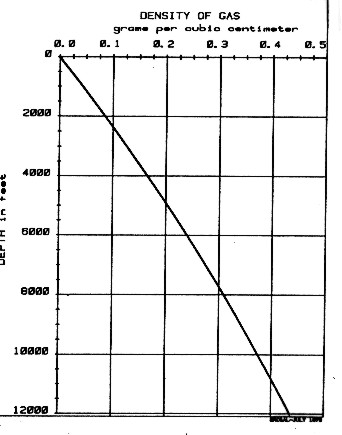Where:
DENS = density of composite material (gm/cc or kg/m3)
DENSH = density of hydrocarbons (gm/cc or kg/m3)
DENSMA = density of matrix rock (gm/cc or kg/m3)
DENSSH = density of shale (gm/cc or kg/m3)
DENSW = density of water (gm/cc or kg/m3)
PHIe = porosity (fractional)
Sw = water saturation (fractional)
Vsh = shale volume (fractional)

This equation is called the Log Response Equation for the density log and forms the basis of density log analysis theory. Its use is discussed more thoroughly in Chapter Eleven. The equation is rigorous and a law of physics.

If a gas analysis or gas density is not available, DENSh for a typical gas in a normal pressure, normal temperature region, such as western Canada, can be chosen from the graph shown at the right.NUMERICAL EXAMPLE
Assume data as follows:
Vsh = 0.10
PHIe = 0.20
Sw = 0.25
DENSSH = 2.60 gm/cc
DENSMA = 2.70 gm/cc
DENSW = 1.00 gm/cc
DENSH = 0.35 gm/cc

DENS = 0.20 * (1 - 0.25) * 0.35
+ 0.20 * 0.25 * 1.00
+ 0.10 * 2.60
+ (1 - 0.10 - 0.20) * 2.60
= 2.055 gm/ccPHYSICS OF DENSITY LOG MEASUREMENT
In-situ formation density is measured by means of gamma ray scattering. A focused beam of gamma rays is emitted from the logging tool into the formation.

Ancient density logs are recorded in gamma ray counts per second. You get to work out the transform to density using a semi-logarithmic High - Low porosity technique as described for the neutron log. Here, high count rate = low density = high porosity. Semi-log crossplots of count rate versus core density or core porosity will calibrate the method. These tools have a single detector and are not compensated for borehole effects. Slim hole versions were widely used in strat holes and in mineral exploration projects. Charts for some specific tools can be found in the literature, such as the one shown below.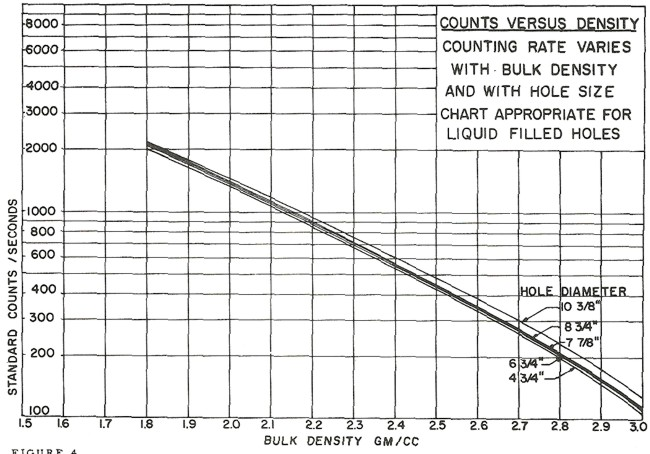Counts per second to density transform for a Schlumberger PGT-A density tool. Each tool iteration and each service company requires a specific chart. Density varies with hole size, mud weight, and mud cake thickness.

These tools are severely affected by hole size, mud weight, mud cake thickness, source type and strength, source detector spacing, and detector efficiency. The High-Low calibration method compensates for all these problems, but available charts do not. In the earliest versions of these tools, the source strength decayed  rapidly, so count rates definitely need to be normalized on a well by well basis.Most density transforms never made it into published chart books. This one did - Schlumberger PGT-C or D density count rates to porosity. Additional charts are available to correct for mud cake thickness and mud weight, and for air-filled holes. The count rate charts appeared in 1966 chart books, well after they were no longer needed, and disappeared after 1968. Most density count rate charts are very hard to find unless you have a good supply of ancient chart books from 1958 through 1968 - a 10 CD set of ancient chart books was published by Denver Well Log Society and sold through SPWLA.

In modern density logging tools, gamma ray detectors measure the gamma ray flux at two or more distances from the source. The more distant detector count rate is converted to a measure of the formation density. The difference between the long spaced and short spaced count rates is converted into a correction factor to compensate for the effects of mudcake and wellbore rugosity.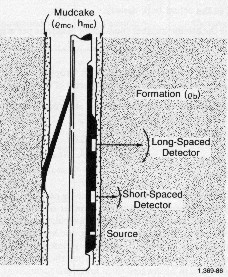The gamma ray source is Cesium137 emitting gamma rays with a fixed energy of 662 KeV. The gamma ray detectors are lithium iodide crystals that emit a photon of light when a gamma ray impacts the crystal. This light flash is amplified by a photo-multiplier that converts light into an electrical pulse which is counted by a digital circuit. The counts are summed over a particular time period to obtain an average counts per second. The count rate is further processed by computer to obtain formation density, according to the method described below.

The source is collimated (or focused) by appropriate shielding, as are the detectors, to prevent direct gamma ray paths through the tool and the borehole. Natural gamma rays from the formation are low energy and filtered from the detectors.

Gamma rays decay exponentially with distance from the source and with the number of scattering sites along their travel path. The gamma ray flux at a detector can be described by:
18: G = Go * e^( –Nsc * Cs * X)

Where
G = gamma ray flux at detector
Go = initial gamma ray flux emitted from the source (cps/cm2)
Nsc = number of scattering centers (electrons/cc)
Cs = scattering cross section of each scattering center (cm2)
X = distance from source to detector (cm)

For the medium energy gamma ray used in logging, the dominant interaction between the source gamma rays and the formation is through Compton scattering. The Compton effect was observed by Arthur Compton in 1923.

Compton scattering or Compton effect, is the decrease in energy (increase in wavelength) of an X-ray or gamma ray photon when it interacts with matter. The amount the wavelength increases is called the Compton shift. Although nuclear Compton scattering exists, Compton scattering usually refers to the interaction involving only the electrons of an atom.

Thus the number of scattering sites, Nsc, is the number of electrons / cc in the material being bombarded by the gamma ray source..

Avogadro’s constant (No) allows us to connect density to the number of nuclei / cm3 and the number of electrons (Nsc). The number of scattering centers per volume can be obtained for a substance from:
19: Nsc = No * DENSe * Z / A

Where
A = atomic weight
Z = number of electrons per atom
DENSe = electron density (g/cc)
No = 6.03 X 10^23

The attenuation expression can be rewritten as:
20: G = Go * e^( – No * DENSe * Z / A * Cs * X)

By solving for DENSe in this equation, we arrive at the basic equation for the density log response.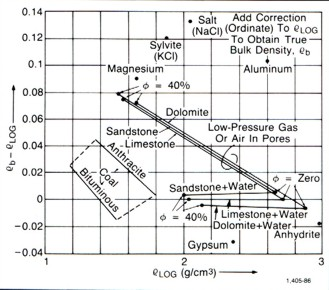In scaling the density log, the transform assumes Z/A = 0.5, which is true for most materials but not for all of them. When Z/A is not 0.5, the DENSe term needs to be corrected.

The correct bulk density (DENS) can be obtained from the density log reading (DENSe) by:
21: DENS = 0.5 * DENSe / (Z/A)

The most glaring example, however, is hydrogen with Z/A = 1.0, which causes the electron density index of water to be 11% larger than its bulk density. This would create problems for porous media were it not for a simple transform proposed many years ago and adopted by all service companies (Gaymard and Poupon, 1968). The density inferred from gamma ray scattering is modified by the following expression
22: DENS = 1.0704 * DENS e – 0.188

This transform of the measured value practically eliminates the problem in porous water-filled sedimentary formations.PHYSICS OF PHOTOELECTRIC LOG MEASUREMENT
The photoelectric effect is a quantum electronic phenomenon in which photoelectrons are emitted from matter after the absorption of energy from electromagnetic radiation such as X-rays or gamma rays. The effect is also termed the Hertz effect, but the term has fallen into disuse. The photoelectric effect was known as early as 1839 and has been studied by many physicists, including Becquerel, Hertz, Tesla, Einstein, and others.

Study of the photoelectric effect led to important steps in understanding the quantum nature of light, and the concept of wave–particle duality. Modern applications of the photoelectric effect include solar panels and CCD image sensors used in digital cameras, and well logging applications.

The probability of photoelectric absorption depends on the gamma ray energy and on the atomic number, Z, of the scattering material. This means that scattering cross section, Cs, of a given material is not a constant, but a function of the gamma ray energy, E. Therefore, a change in detected gamma ray flux could be caused by either a change in sample density or a change in atomic number, or both.

The highest energy gamma rays will carry density information while the lowest will be affected by density and the Z of the scattering medium. Current logging tool design exploits this fact by measuring the energy level of the detected gamma rays as well as their count rate.

A practical unit to describe the Z of a mineral mixture is the photoelectric factor, abbreviated PEF or PE. For a single element of atomic number Z, it is defined as:
23: PE = (Z/10)^3.6.

For any mixture of materials, PE can be computed from the mass-weighted average of the PE values of each element in the mixture.

Cs in equation 18 can now be replaced by:
24: Cs = A * PE + B

Where:
A and B are energy dependent terms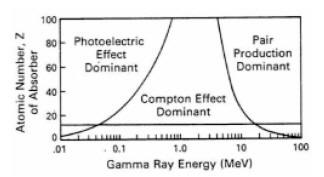The coefficient A, associated with PE, varies as 1/(E^3), whereas the coefficient B, associated with the Compton scattering, is practically constant.

The illustration on the right shows a graph of the probability for Compton scattering or photoelectric absorption, as a function of gamma ray energy and atomic number of the scattering material.

At lower gamma ray energies the photoelectric absorption dominates the process even for sedimentary rocks (which have an average Z between 11 and 16). The horizontal line at 13 corresponds to aluminum, which can be viewed as a suitable proxy for a real rock when dealing with gamma ray scattering.

At very high energy (above 1 MeV) a third process for gamma ray interaction, pair production, becomes available. Pair production is not of any concern for gamma-gamma density devices employing Cs137 sources with gamma ray emission at 662 KeV, well below the pair production threshold.

A measurement technique that compares the propagation of gamma rays at higher and lower energies is used to determine the amount of absorption due to the photoelectric effect, and thus to deduce the PE of the scattering material (rock). The PE of the formation can help distinguish between the common minerals that form sedimentary rocks, or can be used, in conjunction with other data such as density, to determine the composition of mineral mixtures.

However, the use of the PE techniques is compromised by the presence of barite weighted drilling mud. The large Z = 56 of barite makes it a very efficient low-energy gamma ray absorber, so any amount of it in mudcake or in the invasion fluids can seriously alter the apparent PE of the formation, to the point of rendering it useless for interpretation.

Page Views ---- Since 01 Jan 2015
Copyright 2023 by Accessible Petrophysics Ltd.
CPH Logo, "CPH", "CPH Gold Member", "CPH Platinum Member", "Crain's Rules", "Meta/Log", "Computer-Ready-Math", "Petro/Fusion Scripts" are Trademarks of the Author